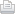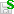Journal cover Journal topic
Nonlinear Processes in Geophysics An interactive open-access journal of the European Geosciences Union
Journal topic

Journal metrics

•IF 1.699
•IF 5-year
1.559
•CiteScore
1.61
•SNIP 0.884
•IPP 1.49
•SJR 0.648
•Scimago H
index 52
•h5-index 21

Abstracted/indexed

Abstracted/indexed
https://doi.org/10.5194/npgd-1-519-2014https://doi.org/10.5194/npgd-1-519-2014Research article 11 Apr 2014

Research article | 11 Apr 2014

Review status
This discussion paper is a preprint. It has been under review for the journal Nonlinear Processes in Geophysics (NPG). The revised manuscript was not accepted.

On the nonlinear feedback loop and energy cycle of the non-dissipative Lorenz model

B.-W. Shen B.-W. Shen
• ESSIC, University of Maryland, College Park, Mesoscale Atmospheric Processes Laboratory, Code 612, NASA Goddard Space Flight Center, Greenbelt, MD 20771, USA

Abstract. In this study, we discuss the role of the nonlinear terms and linear (heating) term in the energy cycle of the three-dimensional (XYZ) non-dissipative Lorenz model (3D-NLM). (X, Y, Z) represent the solutions in the phase space. We first present the closed-form solution to the nonlinear equation d2 X/dτ2+ (X2/2)X = 0, τ is a non-dimensional time, which was never documented in the literature. As the solution is oscillatory (wave-like) and the nonlinear term (X2) is associated with the nonlinear feedback loop, it is suggested that the nonlinear feedback loop may act as a restoring force. We then show that the competing impact of nonlinear restoring force and linear (heating) force determines the partitions of the averaged available potential energy from Y and Z modes, respectively, denoted as APEY and APEZ. Based on the energy analysis, an energy cycle with four different regimes is identified with the following four points: A(X, Y) = (0,0), B = (Xt, Yt), C = (Xm, Ym), and D = (Xt, -Yt). Point A is a saddle point. The initial perturbation (X, Y, Z) = (0, 1, 0) gives (Xt, Yt) = ( 2σr , r) and (Xm, Ym) = (2  σr , 0). σ is the Prandtl number, and r is the normalized Rayleigh number. The energy cycle starts at (near) point A, A+ = (0, 0+) to be specific, goes through B, C, and D, and returns back to A, i.e., A- = (0,0-). From point A to point B, denoted as Leg AB, where the linear (heating) force dominates, the solution X grows gradually with { KE↑, APEY↓, APEZ↓}. KE is the averaged kinetic energy. We use the upper arrow (↑) and down arrow (↓) to indicate an increase and decrease, respectively. In Leg BC (or CD) where nonlinear restoring force becomes dominant, the solution X increases (or decreases) rapidly with {KE↑, APEY↑, APEZ↓} (or {KE↓, APEY↓, APEZ↑}). In Leg DA, the solution X decreases slowly with {KE↓, APEY↑, APEZ↑ }. As point A is a saddle point, the aforementioned cycle may be only half of a "big" cycle, displaying the wing pattern of a glasswinged butterfly, and the other half cycle is antisymmetric with respect to the origin, namely B = (-Xt, -Yt), C = (-Xm, 0), and D = (-Xt, Yt).

B.-W. Shen
Interactive discussion
Status: closed
Status: closed
AC: Author comment | RC: Referee comment | SC: Short comment | EC: Editor comment- Printer-friendly version- Supplement
• RC C94:
• RC C160:
• AC C469:
• AC C537:
• AC C483:
Interactive discussion
Status: closed
Status: closed
AC: Author comment | RC: Referee comment | SC: Short comment | EC: Editor comment- Printer-friendly version- Supplement
• RC C94:
• RC C160:
• AC C469:
• AC C537:
• AC C483:
B.-W. Shen
B.-W. Shen
Viewed
Total article views: 2,658 (including HTML, PDF, and XML)
HTML PDF XML Total BibTeX EndNote
1,927 614 117 2,658 32 42
• HTML: 1,927
• PDF: 614
• XML: 117
• Total: 2,658
• BibTeX: 32
• EndNote: 42